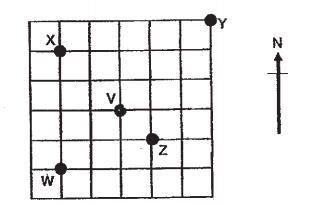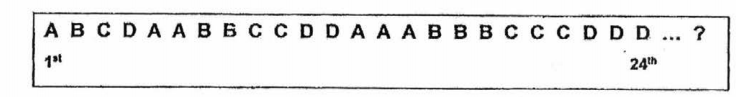# Primary 5 CA1 Practice #3

Time elapsed:
Q1. The common multiples of two 1-digit numbers are 16, 24 and 40. What is the largest possible values of these two 1-digit numbers?     [2 marks]
Q2. It takes 5 112 hours to drive to Kuala Lumpur. Amanda's father has already driven 3 34 hours. How much longer will he have to drive before he arrives in Kuala Lumpur?     [2 marks]
Q3. Find the value of 4 + 6 x (501 - 51).     [1 mark]
Q4. A shop sold 40 similar light bulbs for \$640. What was the price of each light bulb?     [1 mark]
Q5. When rounded off to the nearest dollar, a carton of mandarin oranges cost \$36. Which one of the following could be the actual cost of the carton of mandarin oranges?     [1 mark]
Q6. What is the difference between 2 23 and 14?     [2 marks]
Q7. How many of the letters have at least a line of symmetry?     [1 mark]Q8. There were 200 people at a fun fair. 120 were children and the rest were adults. 38 of the adults were men. How many women were there?     [2 marks]
Q9. Which of the following decimals is nearest to 1?     [1 mark]
Q10. 9 is a common factor of ___     [1 mark]
Q11. Find the product of 14 and 89     [1 mark]
Q12. Kate ran 79 km. Raymond ran 23 km less than Kate. How far did Raymond run?     [1 mark]
Q13. Which one of the following are common factors of 36 and 90?     [1 mark]
Q14. Express 7.04 as a fraction.     [1 mark]
Q15. Javier is thinking of a 7-digit number. The digit in the millions place is twice the digit in the ten thousands place. Which one of the following numbers is Javier thinking of?     [1 mark]
Q16. Raymond is at Point V and facing north-east. Which point will he face after making a 3/4-turn clockwise?     [1 mark]Q17. The following pattern is formed using 4 letters, A, B, C and D. What is the 60th letter?     [2 marks]Q18. What is the value of the digit 7 in 1 070 003?     [1 mark]
Q19. Find the product of 437 and 68.     [2 marks]
Q20. Vincent bought 2 pies. When he reached home, he gave all the pies away to his 4 sisters to share equally. What fraction of the pie did each sister receive?     [2 marks]